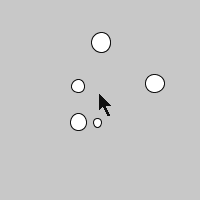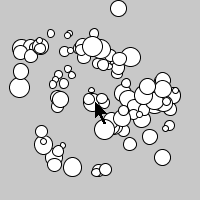# Flyers

example processing creating-classes animation

This program creates a `Flyer` class to encapsulate all of the logic for creating a “bug”that follows the mouse around using a heading angle and a speed. This provides more realistic movement, but it lets us keep our `draw()` function nice and simple.

``````Flyer[] flyers = new Flyer;

void setup(){
size(200, 200);

for(int i = 0; i < flyers.length; i++){
flyers[i] = new Flyer();
}
}

void draw(){
background(200);

for(int i = 0; i < flyers.length; i++){
flyers[i].step();
flyers[i].draw();
}
}

class Flyer{

float x = random(width);
float y = random(height);
float speed = random(1, 3);

void step(){

float angleToMouse = atan2(mouseY-y, mouseX-x);

//prevent case where heading is 350 and angleToMouse is 10
angleToMouse += TWO_PI;
}
angleToMouse -= TWO_PI;
}

//turn towards mouse
}
else{
}

//move in direction

//wrap around edges
if(x < 0){
x = width;
}
if(x > width){
x = 0;
}

if(y < 0){
y = height;
}
if(y > height){
y = 0;
}

}

void draw(){
}

}
``````Code Editor

See the Pen by Happy Coding (@KevinWorkman) on CodePen.

If this seems complicated, think about it this way: when using a class, we only need to worry about the behavior of a single `Flyer` instance (in other words, we only need to know how a single “bug” behaves). Then when we get that single `Flyer` working, we can use an array or an `ArrayList` to add as many `Flyer` instances as we want!## Tweak Ideas

• Give each `Flyer` a random color.
• Move the call to `background()` to the `setup()` function to show trails.
• Make them run away from the mouse instead of chase it.